# Which Australian bank offers the highest interest rates under the term deposits for 1 year06.07.2020

## Bankchart.com.au has surveyed the terms of term deposits for 1 year in the national currency. The survey covered all the deposits with any form of interests repayment, regardless of the replenishment option or the deposit partial withdrawal option. Our website's analysts have studied the loan offers from all Australian banks. Review date - 06.07.2020

As of 23 June 2020 the deposits under review have been offered by 32 banks.  The average interest rate under such deposits equals 0,97 % p.a. The total number of the deposits programs available for the specified terms is 118.

The maximal return among the deposits under review is offered by Arab Bank. The interest rate under "Fixed Term Deposit" deposit program constitutes 1,60 % p.a. The table below shows the best offers by each bank selected by Bankchart.com.au (in terms of profitability) for the aforementioned terms.

### The TOP-32 term deposits for 1 year, as of 23 June 2020

#

Name

Interest Rate, %

1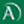Arab Bank

«Fixed Term Deposit»

1,60

interest rate, %

rate type

fixed

interest periodicity

at maturity

minimum sum

\$ 50 000,00

2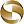Mega International Commercial Bank

«Term Deposits»

1,50

interest rate, %

rate type

fixed

interest periodicity

annually

minimum sum

\$ 1 000,00

3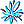AMP Bank

«Term Deposits»

1,40

interest rate, %

rate type

fixed

interest periodicity

at maturity

minimum sum

\$ 25 000,00

4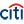Citibank

«Term deposit»

1,35

interest rate, %

rate type

fixed

interest periodicity

monthly

minimum sum

\$ 10 000,00

5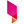Bank Australia

«Term Deposits»

1,35

interest rate, %

rate type

fixed

interest periodicity

at maturity

minimum sum

\$ 500,00

6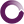Beyond Bank Australia

«Term Deposits»

1,30

interest rate, %

rate type

fixed

interest periodicity

at maturity

minimum sum

\$ 200 000,00

7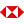HSBC Bank

«Term Deposits»

1,30

interest rate, %

rate type

fixed

interest periodicity

at maturity, annually

minimum sum

\$ 5 000,00

8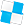Police Bank

«Term Deposits»

1,30

interest rate, %

rate type

fixed

interest periodicity

at maturity

minimum sum

\$ 10 000,00

9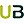Ubank

«Term deposit»

1,30

interest rate, %

rate type

variable

interest periodicity

at maturity

minimum sum

\$ 0,00

10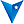BankVic

«Term deposit»

1,25

interest rate, %

rate type

fixed

interest periodicity

at maturity

minimum sum

\$ 25 000,00

11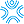Bank First

«Term Deposits»

1,25

interest rate, %

rate type

fixed

interest periodicity

monthly

minimum sum

\$ 50 000,00

12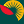Suncorp Bank

«Term Deposit»

1,20

interest rate, %

rate type

fixed

interest periodicity

at maturity

minimum sum

\$ 1 000 000,00

13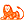ING Direct

«Personal Term Deposit»

1,15

interest rate, %

rate type

fixed

interest periodicity

at maturity

minimum sum

\$ 10 000,00

14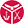Defence Bank

«Defence Bank Super Term Deposits»

1,15

interest rate, %

rate type

fixed

interest periodicity

at maturity

minimum sum

\$ 10 000,00

15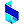Bank of Sydney

«Online Term Deposit»

1,15

interest rate, %

rate type

fixed

interest periodicity

at maturity

minimum sum

\$ 1 000,00

16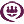RACQ

«Term Deposit»

1,15

interest rate, %

rate type

fixed

interest periodicity

monthly

minimum sum

\$ 1 000,00

17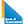Bank of Queensland

«Term deposit»

1,10

interest rate, %

rate type

fixed

interest periodicity

at maturity

minimum sum

\$ 5 000,00

18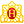Heritage Bank

«Term Deposits»

1,10

interest rate, %

rate type

fixed

interest periodicity

at maturity

minimum sum

\$ 1 000,00

19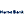Hume Bank

«Term Deposits»

1,10

interest rate, %

rate type

fixed

interest periodicity

at maturity, annually

minimum sum

\$ 5 000,00

20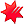National Australia Bank

«NAB Term Deposits»

1,10

interest rate, %

rate type

fixed

interest periodicity

at maturity

minimum sum

\$ 5 000,00

21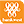Bank of Western Australia

«Online Term Deposit»

1,05

interest rate, %

rate type

fixed

interest periodicity

quarterly, compound 6 monthly

minimum sum

\$ 5 000,00

22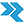Rural Bank

«Long Term Deposit»

1,05

interest rate, %

rate type

fixed

interest periodicity

annually

minimum sum

\$ 50 000,00

23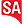Bank SA

«Term Deposits»

1,05

interest rate, %

rate type

fixed

interest periodicity

at maturity

minimum sum

\$ 1 000,00

24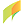G&C Mutual Bank

«Term Deposits»

1,00

interest rate, %

rate type

fixed

interest periodicity

at maturity, annually

minimum sum

\$ 1 000,00

25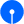State Bank of India

«Term Deposits»

1,00

interest rate, %

rate type

fixed

interest periodicity

at maturity

minimum sum

\$ 100,00

26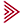Bendigo and Adelaide Bank

«Term deposit»

1,00

interest rate, %

rate type

fixed

interest periodicity

at maturity

minimum sum

\$ 5 000,00

27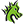St. George Bank

«Term Deposits»

0,90

interest rate, %

rate type

fixed

interest periodicity

at maturity, half yearly

minimum sum

\$ 1 000,00

28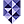Bank of Melbourne

«Term Deposits»

0,90

interest rate, %

rate type

fixed

interest periodicity

at maturity, half yearly

minimum sum

\$ 1 000,00

29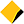Commonwealth Bank of Australia

«Term Deposits»

0,90

interest rate, %

rate type

fixed

interest periodicity

annually

minimum sum

\$ 50 000,00

30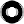Macquarie Bank

«Term Deposit»

0,90

interest rate, %

rate type

fixed

interest periodicity

monthly

minimum sum

\$ 0,00

31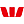Westpac Bank

«Term Deposit»

0,85

interest rate, %

rate type

fixed

interest periodicity

at maturity, annually

minimum sum

\$ 5 000,00

32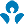Australia and New Zealand (ANZ)

0,85

interest rate, %

rate type

fixed

interest periodicity

quarterly

minimum sum

\$ 5 000,00

To view the up-to-date rating of the deposits at our website here.